Open in App
Not now

# GUI chat application using Tkinter in Python

• Difficulty Level : Medium
• Last Updated : 11 Sep, 2022

Chatbots are computer program that allows user to interact using input methods. The Application of chatbots is to interact with customers in big enterprises or companies and resolve their queries.  Chatbots are mainly built for answering standard FAQs.

The benefit of this type of system is that customer is no longer required to follow the same traditional mean of communication Instead they can just chat with the bot and can resolve their query.

Example 1) This is a simple GUI (Graphical User Interface) chat application where users can interact with the bot.

Prerequisites: Tkinter

`pip install tkinter`

Example

## Python

 `from` `tkinter ``import` `*`   `# GUI` `root ``=` `Tk()` `root.title(``"Chatbot"``)`   `BG_GRAY ``=` `"#ABB2B9"` `BG_COLOR ``=` `"#17202A"` `TEXT_COLOR ``=` `"#EAECEE"`   `FONT ``=` `"Helvetica 14"` `FONT_BOLD ``=` `"Helvetica 13 bold"`   `# Send function` `def` `send():` `    ``send ``=` `"You -> "` `+` `e.get()` `    ``txt.insert(END, ``"\n"` `+` `send)`   `    ``user ``=` `e.get().lower()`   `    ``if` `(user ``=``=` `"hello"``):` `        ``txt.insert(END, ``"\n"` `+` `"Bot -> Hi there, how can I help?"``)`   `    ``elif` `(user ``=``=` `"hi"` `or` `user ``=``=` `"hii"` `or` `user ``=``=` `"hiiii"``):` `        ``txt.insert(END, ``"\n"` `+` `"Bot -> Hi there, what can I do for you?"``)`   `    ``elif` `(user ``=``=` `"how are you"``):` `        ``txt.insert(END, ``"\n"` `+` `"Bot -> fine! and you"``)`   `    ``elif` `(user ``=``=` `"fine"` `or` `user ``=``=` `"i am good"` `or` `user ``=``=` `"i am doing good"``):` `        ``txt.insert(END, ``"\n"` `+` `"Bot -> Great! how can I help you."``)`   `    ``elif` `(user ``=``=` `"thanks"` `or` `user ``=``=` `"thank you"` `or` `user ``=``=` `"now its my time"``):` `        ``txt.insert(END, ``"\n"` `+` `"Bot -> My pleasure !"``)`   `    ``elif` `(user ``=``=` `"what do you sell"` `or` `user ``=``=` `"what kinds of items are there"` `or` `user ``=``=` `"have you something"``):` `        ``txt.insert(END, ``"\n"` `+` `"Bot -> We have coffee and tea"``)`   `    ``elif` `(user ``=``=` `"tell me a joke"` `or` `user ``=``=` `"tell me something funny"` `or` `user ``=``=` `"crack a funny line"``):` `        ``txt.insert(` `            ``END, ``"\n"` `+` `"Bot -> What did the buffalo say when his son left for college? Bison.! "``)`   `    ``elif` `(user ``=``=` `"goodbye"` `or` `user ``=``=` `"see you later"` `or` `user ``=``=` `"see yaa"``):` `        ``txt.insert(END, ``"\n"` `+` `"Bot -> Have a nice day!"``)`   `    ``else``:` `        ``txt.insert(END, ``"\n"` `+` `"Bot -> Sorry! I didn't understand that"``)`   `    ``e.delete(``0``, END)`     `lable1 ``=` `Label(root, bg``=``BG_COLOR, fg``=``TEXT_COLOR, text``=``"Welcome"``, font``=``FONT_BOLD, pady``=``10``, width``=``20``, height``=``1``).grid(` `    ``row``=``0``)`   `txt ``=` `Text(root, bg``=``BG_COLOR, fg``=``TEXT_COLOR, font``=``FONT, width``=``60``)` `txt.grid(row``=``1``, column``=``0``, columnspan``=``2``)`   `scrollbar ``=` `Scrollbar(txt)` `scrollbar.place(relheight``=``1``, relx``=``0.974``)`   `e ``=` `Entry(root, bg``=``"#2C3E50"``, fg``=``TEXT_COLOR, font``=``FONT, width``=``55``)` `e.grid(row``=``2``, column``=``0``)`   `send ``=` `Button(root, text``=``"Send"``, font``=``FONT_BOLD, bg``=``BG_GRAY,` `              ``command``=``send).grid(row``=``2``, column``=``1``)`   `root.mainloop()`

### Output: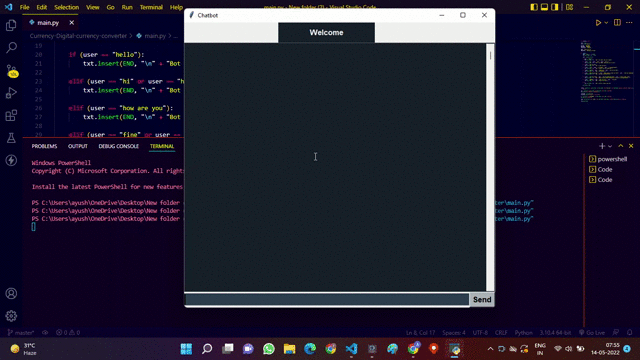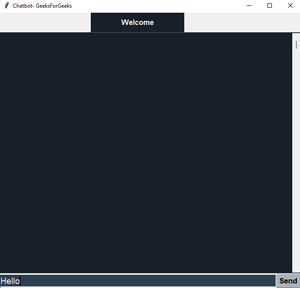Example 2: This is a simple GUI (Graphical User Interface) chat application where multiple users can connect with each other in a client-server architecture i.e the clients will interact with the help of the server.

Prerequisites: Tkinter, Socket Programming, and Multithreading

Server Side Script:  This script file name is ‘client.py'(say). Since only the clients will interact the server script does not have a GUI.

Example

## Python3

 `# import socket library` `import` `socket`   `# import threading library` `import` `threading`   `# Choose a port that is free` `PORT ``=` `5000`   `# An IPv4 address is obtained` `# for the server.` `SERVER ``=` `socket.gethostbyname(socket.gethostname())`   `# Address is stored as a tuple` `ADDRESS ``=` `(SERVER, PORT)`   `# the format in which encoding` `# and decoding will occur` `FORMAT` `=` `"utf-8"`   `# Lists that will contains` `# all the clients connected to` `# the server and their names.` `clients, names ``=` `[], []`   `# Create a new socket for` `# the server` `server ``=` `socket.socket(socket.AF_INET,` `                       ``socket.SOCK_STREAM)`   `# bind the address of the` `# server to the socket` `server.bind(ADDRESS)`   `# function to start the connection`     `def` `startChat():`   `    ``print``(``"server is working on "` `+` `SERVER)`   `    ``# listening for connections` `    ``server.listen()`   `    ``while` `True``:`   `        ``# accept connections and returns` `        ``# a new connection to the client` `        ``#  and  the address bound to it` `        ``conn, addr ``=` `server.accept()` `        ``conn.send(``"NAME"``.encode(``FORMAT``))`   `        ``# 1024 represents the max amount` `        ``# of data that can be received (bytes)` `        ``name ``=` `conn.recv(``1024``).decode(``FORMAT``)`   `        ``# append the name and client` `        ``# to the respective list` `        ``names.append(name)` `        ``clients.append(conn)`   `        ``print``(f``"Name is :{name}"``)`   `        ``# broadcast message` `        ``broadcastMessage(f``"{name} has joined the chat!"``.encode(``FORMAT``))`   `        ``conn.send(``'Connection successful!'``.encode(``FORMAT``))`   `        ``# Start the handling thread` `        ``thread ``=` `threading.Thread(target``=``handle,` `                                  ``args``=``(conn, addr))` `        ``thread.start()`   `        ``# no. of clients connected` `        ``# to the server` `        ``print``(f``"active connections {threading.activeCount()-1}"``)`   `# method to handle the` `# incoming messages`     `def` `handle(conn, addr):`   `    ``print``(f``"new connection {addr}"``)` `    ``connected ``=` `True`   `    ``while` `connected:` `          ``# receive message` `        ``message ``=` `conn.recv(``1024``)`   `        ``# broadcast message` `        ``broadcastMessage(message)`   `    ``# close the connection` `    ``conn.close()`   `# method for broadcasting` `# messages to the each clients`     `def` `broadcastMessage(message):` `    ``for` `client ``in` `clients:` `        ``client.send(message)`     `# call the method to` `# begin the communication` `startChat()`

Client-Side Script:

## Python3

 `# import all the required  modules` `import` `socket` `import` `threading` `from` `tkinter ``import` `*` `from` `tkinter ``import` `font` `from` `tkinter ``import` `ttk`   `# import all functions /` `#  everything from chat.py file` `from` `chat ``import` `*`   `PORT ``=` `5050` `SERVER ``=` `"192.168.0.103"` `ADDRESS ``=` `(SERVER, PORT)` `FORMAT` `=` `"utf-8"`   `# Create a new client socket` `# and connect to the server` `client ``=` `socket.socket(socket.AF_INET,` `                       ``socket.SOCK_STREAM)` `client.connect(ADDRESS)`     `# GUI class for the chat` `class` `GUI:` `    ``# constructor method` `    ``def` `__init__(``self``):`   `        ``# chat window which is currently hidden` `        ``self``.Window ``=` `Tk()` `        ``self``.Window.withdraw()`   `        ``# login window` `        ``self``.login ``=` `Toplevel()` `        ``# set the title` `        ``self``.login.title(``"Login"``)` `        ``self``.login.resizable(width``=``False``,` `                             ``height``=``False``)` `        ``self``.login.configure(width``=``400``,` `                             ``height``=``300``)` `        ``# create a Label` `        ``self``.pls ``=` `Label(``self``.login,` `                         ``text``=``"Please login to continue"``,` `                         ``justify``=``CENTER,` `                         ``font``=``"Helvetica 14 bold"``)`   `        ``self``.pls.place(relheight``=``0.15``,` `                       ``relx``=``0.2``,` `                       ``rely``=``0.07``)` `        ``# create a Label` `        ``self``.labelName ``=` `Label(``self``.login,` `                               ``text``=``"Name: "``,` `                               ``font``=``"Helvetica 12"``)`   `        ``self``.labelName.place(relheight``=``0.2``,` `                             ``relx``=``0.1``,` `                             ``rely``=``0.2``)`   `        ``# create a entry box for` `        ``# tyoing the message` `        ``self``.entryName ``=` `Entry(``self``.login,` `                               ``font``=``"Helvetica 14"``)`   `        ``self``.entryName.place(relwidth``=``0.4``,` `                             ``relheight``=``0.12``,` `                             ``relx``=``0.35``,` `                             ``rely``=``0.2``)`   `        ``# set the focus of the cursor` `        ``self``.entryName.focus()`   `        ``# create a Continue Button` `        ``# along with action` `        ``self``.go ``=` `Button(``self``.login,` `                         ``text``=``"CONTINUE"``,` `                         ``font``=``"Helvetica 14 bold"``,` `                         ``command``=``lambda``: ``self``.goAhead(``self``.entryName.get()))`   `        ``self``.go.place(relx``=``0.4``,` `                      ``rely``=``0.55``)` `        ``self``.Window.mainloop()`   `    ``def` `goAhead(``self``, name):` `        ``self``.login.destroy()` `        ``self``.layout(name)`   `        ``# the thread to receive messages` `        ``rcv ``=` `threading.Thread(target``=``self``.receive)` `        ``rcv.start()`   `    ``# The main layout of the chat` `    ``def` `layout(``self``, name):`   `        ``self``.name ``=` `name` `        ``# to show chat window` `        ``self``.Window.deiconify()` `        ``self``.Window.title(``"CHATROOM"``)` `        ``self``.Window.resizable(width``=``False``,` `                              ``height``=``False``)` `        ``self``.Window.configure(width``=``470``,` `                              ``height``=``550``,` `                              ``bg``=``"#17202A"``)` `        ``self``.labelHead ``=` `Label(``self``.Window,` `                               ``bg``=``"#17202A"``,` `                               ``fg``=``"#EAECEE"``,` `                               ``text``=``self``.name,` `                               ``font``=``"Helvetica 13 bold"``,` `                               ``pady``=``5``)`   `        ``self``.labelHead.place(relwidth``=``1``)` `        ``self``.line ``=` `Label(``self``.Window,` `                          ``width``=``450``,` `                          ``bg``=``"#ABB2B9"``)`   `        ``self``.line.place(relwidth``=``1``,` `                        ``rely``=``0.07``,` `                        ``relheight``=``0.012``)`   `        ``self``.textCons ``=` `Text(``self``.Window,` `                             ``width``=``20``,` `                             ``height``=``2``,` `                             ``bg``=``"#17202A"``,` `                             ``fg``=``"#EAECEE"``,` `                             ``font``=``"Helvetica 14"``,` `                             ``padx``=``5``,` `                             ``pady``=``5``)`   `        ``self``.textCons.place(relheight``=``0.745``,` `                            ``relwidth``=``1``,` `                            ``rely``=``0.08``)`   `        ``self``.labelBottom ``=` `Label(``self``.Window,` `                                 ``bg``=``"#ABB2B9"``,` `                                 ``height``=``80``)`   `        ``self``.labelBottom.place(relwidth``=``1``,` `                               ``rely``=``0.825``)`   `        ``self``.entryMsg ``=` `Entry(``self``.labelBottom,` `                              ``bg``=``"#2C3E50"``,` `                              ``fg``=``"#EAECEE"``,` `                              ``font``=``"Helvetica 13"``)`   `        ``# place the given widget` `        ``# into the gui window` `        ``self``.entryMsg.place(relwidth``=``0.74``,` `                            ``relheight``=``0.06``,` `                            ``rely``=``0.008``,` `                            ``relx``=``0.011``)`   `        ``self``.entryMsg.focus()`   `        ``# create a Send Button` `        ``self``.buttonMsg ``=` `Button(``self``.labelBottom,` `                                ``text``=``"Send"``,` `                                ``font``=``"Helvetica 10 bold"``,` `                                ``width``=``20``,` `                                ``bg``=``"#ABB2B9"``,` `                                ``command``=``lambda``: ``self``.sendButton(``self``.entryMsg.get()))`   `        ``self``.buttonMsg.place(relx``=``0.77``,` `                             ``rely``=``0.008``,` `                             ``relheight``=``0.06``,` `                             ``relwidth``=``0.22``)`   `        ``self``.textCons.config(cursor``=``"arrow"``)`   `        ``# create a scroll bar` `        ``scrollbar ``=` `Scrollbar(``self``.textCons)`   `        ``# place the scroll bar` `        ``# into the gui window` `        ``scrollbar.place(relheight``=``1``,` `                        ``relx``=``0.974``)`   `        ``scrollbar.config(command``=``self``.textCons.yview)`   `        ``self``.textCons.config(state``=``DISABLED)`   `    ``# function to basically start the thread for sending messages` `    ``def` `sendButton(``self``, msg):` `        ``self``.textCons.config(state``=``DISABLED)` `        ``self``.msg ``=` `msg` `        ``self``.entryMsg.delete(``0``, END)` `        ``snd ``=` `threading.Thread(target``=``self``.sendMessage)` `        ``snd.start()`   `    ``# function to receive messages` `    ``def` `receive(``self``):` `        ``while` `True``:` `            ``try``:` `                ``message ``=` `client.recv(``1024``).decode(``FORMAT``)`   `                ``# if the messages from the server is NAME send the client's name` `                ``if` `message ``=``=` `'NAME'``:` `                    ``client.send(``self``.name.encode(``FORMAT``))` `                ``else``:` `                    ``# insert messages to text box` `                    ``self``.textCons.config(state``=``NORMAL)` `                    ``self``.textCons.insert(END,` `                                         ``message``+``"\n\n"``)`   `                    ``self``.textCons.config(state``=``DISABLED)` `                    ``self``.textCons.see(END)` `            ``except``:` `                ``# an error will be printed on the command line or console if there's an error` `                ``print``(``"An error occurred!"``)` `                ``client.close()` `                ``break`   `    ``# function to send messages` `    ``def` `sendMessage(``self``):` `        ``self``.textCons.config(state``=``DISABLED)` `        ``while` `True``:` `            ``message ``=` `(f``"{self.name}: {self.msg}"``)` `            ``client.send(message.encode(``FORMAT``))` `            ``break`     `# create a GUI class object` `g ``=` `GUI()`

OUTPUT :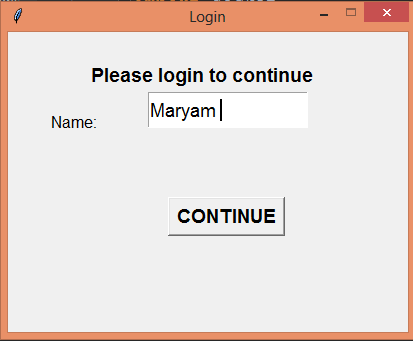CLIENT 1: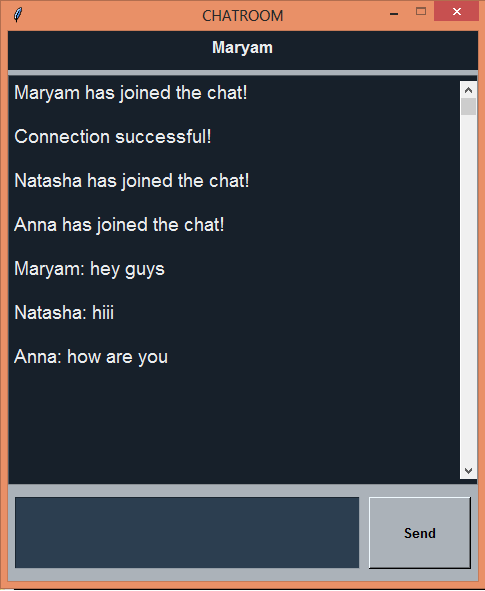CLIENT 2: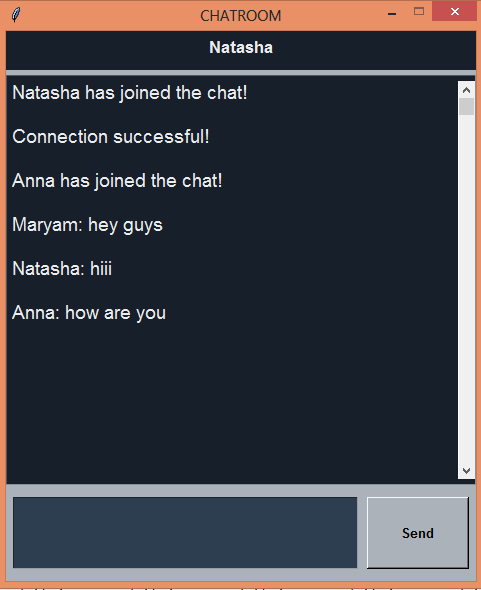CLIENT 3: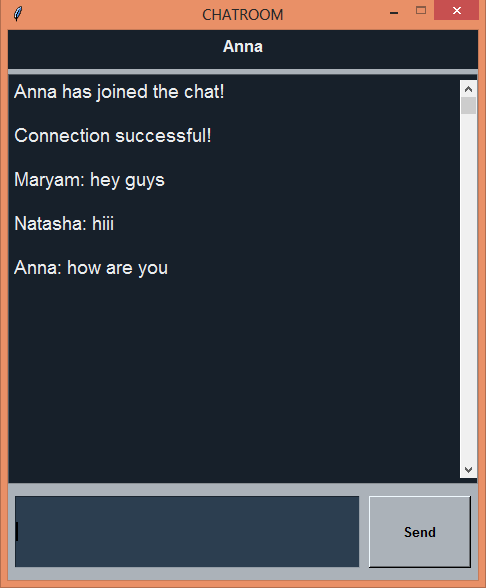SERVER: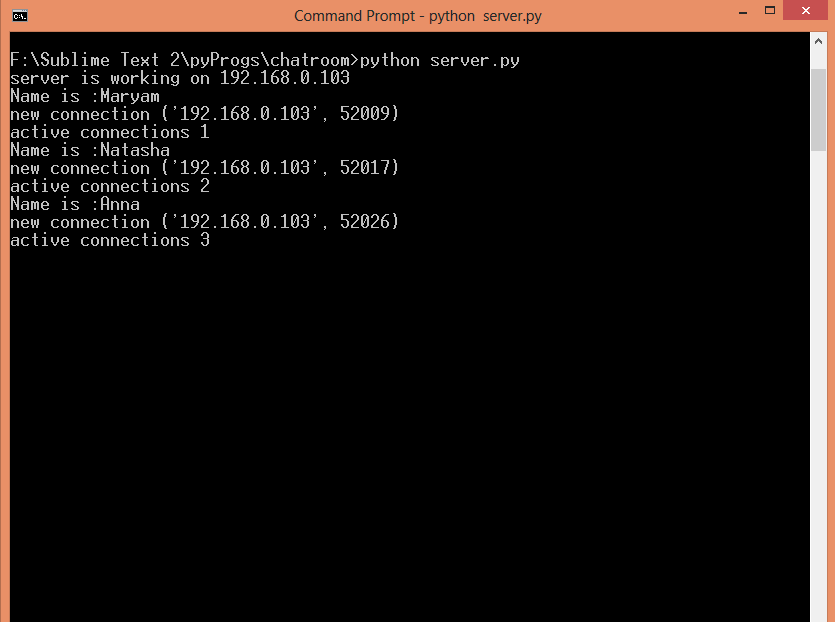My Personal Notes arrow_drop_up
Related Articles